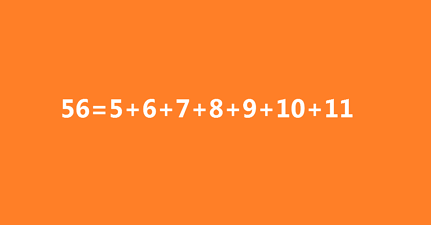# Wayward timesLet $S$ be the set of positive integers, that cannot be written as a sum of at least 2 consecutive positive integers.

For example 10 can be written as: $10 = 1+2+3+4$.

1 cannot be expressed in such a way and thus is in the $S$.

Now build the multiplicative inverse of all the members of $S$, add them all up and then type the result in your answer field.

×

Problem Loading...

Note Loading...

Set Loading...